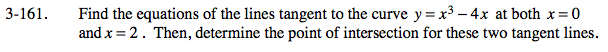### Home > CALC > Chapter Ch3 > Lesson 3.4.2 > Problem3-161

3-161.To find the equation of a tangent line:

Find the slope by evaluating the derivative function at x = 0 or x = 2.

Find the points by evaluating the original function at x = 0 or x = 2.

Write each equation using point-slope form.

To find the point of intersection:

Using the two tangent lines, write and solve a system of equations.

Use the eTool below to visualize this problem.
Click the link at right for the full version of the eTool: Calc 3-161 HW eTool